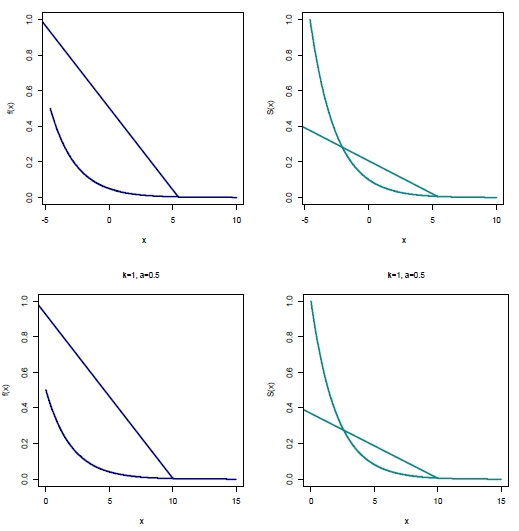# The Discrete Exponentiated Pareto Distribution: Its Properties and Application

• Yadapa Chotedelok Faculty of Science, Kasetsart University
• Winai Bodhisuwan Faculty of Science, Kasetsart University
Keywords: exponentiated Pareto distribution, discretization method, survival function, maximum likelihood estimate

### Abstract

The discrete exponentiated Pareto (DEP) distribution is developed by using the discretization method based on the survival function. It is discretized from the exponentiated Pareto distribution. In this paper, a probability mass function of the DEP distribution is derived. Some mathematical properties and model parameters estimation are discussed. In addition, we applied the DEP distribution to two real datasets. The results of model fitting of these datasets based on the DEP distribution are reasonably constructive. The proposed distribution performs well with a goodness of fit test and some criterions. The distribution can be used as an alternative model for discrete data analytics.